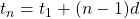# Arithmetic Series

A series is the sum of the terms of a sequence.refers to the sum of the firstterms of a sequence.

The formula for calculating the sum of an arithmetic progression is similar to the formula to find the area of a trapezoid.

The trapezoid-type shape below is made from the terms of an arithmetic sequence. Move the slider to see how the trapezoid-type shape rearranges to a rectangle.

Notice that that the base of the rectangle is calculated as the average of the first and the last term. The height is the number of terms in the sequence.

# Fit two trapezoids together, then divide by 2

Another way to consider this sum is to create a second trapeziod-like shape with the terms of the sequence. The second shape is congruent to the first and fits like a puzzle to form a larger rectangle:

Now we create an area that is double the required sum. We see that the height of the rectangle is the number of terms in the sequence, in this case 7, and the base of the rectangle is the first term plus the last term.

We calculate that double the sum of the first seven terms is:Divide both sides by 2 to calculate the sum required:# Historical note

A mathematician named Karl Frederich Gauss has been called ‘the prince of mathematics’ – due to his many contributions to mathematics. While still a primary school child, he impressed his teachers by calculating that. His teacher had given the class the task, expecting it to take some time. But with Gauss in the room, the task was over in seconds! The website www.nrich.maths.org has a nicely written article on this story.

# Reversing the sequence

Consider the sequence that begins:Calculate the sum of the first 8 terms.Write the sequence a second time and reverse the order of terms:Adding the terms pairwise, we see that we get eight numbers that are equal.Therefore,# Write the process as a formula

We can generalise the first line,toNow as the left hand side is double the number we require, let’s divide by 2:Finally, let’s replace the number of terms – 8 in our example – with.Which can be remembered as ‘first term plus last term, multiply by the number of terms, divided by 2’.

# A little more algebra

Notice, in our formula, we see the value ‘‘. We know thatcan be calculated with the formula.

We can pick out the termfrom the formula, and simply replace it with its calculation:. Then simplify:This is often written as# Practice

## Short Comprehension Exercises

The two formula can be used interchangeably, however you can save yourself one line of work if you use the formula that requires the information you have. That is, the formulais most useful when you have the first term, the last term, and the number of terms. The formulais most useful when you have the first term, the common difference, and the number of terms.

Using either of these formulae, calculate:

1. The sum of the first 12 positive multiples of 5. (That is, 5, 10, 15 … 55, 60).
2. The sum of the first 20 terms of the arithmetic sequence beginning 9, 16, 23 …
3. The sum of the first 41 terms of the arithmetic sequence beginning 80, 76, 72 …

## More Difficult Question

The sum of an arithmetic progression is given by the formula. Find the fifth term of the sequence.

# More Practice

CEMC courseware: Lesson 5 arithmetic series

CK12 Practice Test

Next: Geometric Sequences